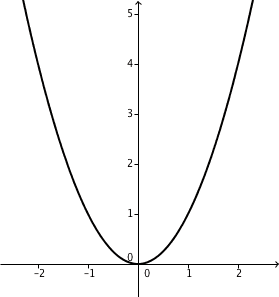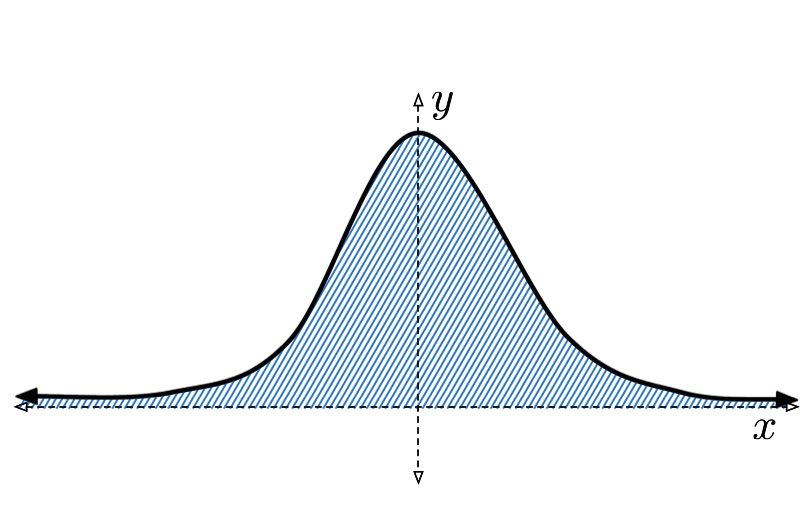Calculus

# Calculus Warmups: Level 4 ChallengesThe ends of a stiff bar $\overline{AB}$ of length 4 slide freely inside a parabolic track (specifically, the parabola $y=x^2$). As they do, the midpoint $M$ of that bar traces a curve. Find the area of the region between the parabola and the curve traced by $M$.

Assume that the bar slides infinitely in both directions.

$L_n=\lim_{x\to\infty}((x+a_1)(x+a_2)\cdots(x+a_n))^{\frac{1}{n}}-x,$

where $\large a_i = \frac{1}{2^i}$.

Find $\displaystyle{\lim_{n\to\infty} (nL_n)}$.

$\sum_{n=0}^{\infty} \frac{1}{(4n)!}$

The above sum can be expressed in the form

$\frac{1}{n} \left( \sum_{k=1}^{mn} e^{i^{k}} \right)$

where $i$ is the imaginary unit and $n$ is some positive multiple of 4.

Find $m$.$\large \int_{- \infty} ^ \infty \frac { e^{2x} - e^x } { x ( e^{2x}+1)( e^x+1) } \, dx$

The integral above has a closed form. Find this closed form.

Give your answer to 3 decimal places.

When $n$ is a positive integer, what is the value of

$\lim_{n \rightarrow \infty} ( -1 ) ^{n-1} \sin ( \pi \sqrt{ n^2 + 0.5 n + 1 } ) ?$

×

Problem Loading...

Note Loading...

Set Loading...